# Latitude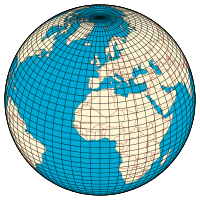A graticule on the Earth as a sphere or an ellipsoid. The lines from pole to pole are lines of constant longitude, or meridians. The circles parallel to the equator are lines of constant latitude, or parallels. The graticule shows the latitude and longitude of points on the surface. In this example meridians are spaced at 6° intervals and parallels at 4° intervals.

In geography, latitude is a geographic coordinate that specifies the north–south position of a point on the Earth's surface. Latitude is an angle (defined below) which ranges from 0° at the Equator to 90° (North or South) at the poles. Lines of constant latitude, or parallels, run east–west as circles parallel to the equator. Latitude is used together with longitude to specify the precise location of features on the surface of the Earth.

## Approximations employed

Two levels of abstraction are employed in the definition of these coordinates. In the first step the physical surface is modelled by the geoid, a surface which approximates the mean sea level over the oceans and its continuation under the land masses. The second step is to approximate the geoid by a mathematically simpler reference surface. The simplest choice for the reference surface is a sphere, but the geoid is more accurately modelled by an ellipsoid. The definitions of latitude and longitude on such reference surfaces are detailed in the following sections. Lines of constant latitude and longitude together constitute a graticule on the reference surface. The latitude of a point on the actual surface is that of the corresponding point on the reference surface, the correspondence being along the normal to the reference surface which passes through the point on the physical surface. Latitude and longitude together with some specification of height constitute a geographic coordinate system as defined in the specification of the ISO 19111 standard.[lower-alpha 1]

Since there are many different reference ellipsoids, the precise latitude of a feature on the surface is not unique: this is stressed in the ISO standard which states that "without the full specification of the coordinate reference system, coordinates (that is latitude and longitude) are ambiguous at best and meaningless at worst". This is of great importance in accurate applications, such as a Global Positioning System (GPS), but in common usage, where high accuracy is not required, the reference ellipsoid is not usually stated.

In English texts the latitude angle, defined below, is usually denoted by the Greek lower-case letter phi (φ or ϕ). It is measured in degrees, minutes and seconds or decimal degrees, north or south of the equator.

The precise measurement of latitude requires an understanding of the gravitational field of the Earth, either to set up theodolites or to determine GPS satellite orbits. The study of the figure of the Earth together with its gravitational field is the science of geodesy. These topics are not discussed in this article. (See for example the textbooks by Torge and Hofmann-Wellenhof and Moritz.)

This article relates to coordinate systems for the Earth: it may be extended to cover the Moon, planets and other celestial objects by a simple change of nomenclature.

The following lists are available:

## History of latitude measurements

The Greeks studied the results of the measurements by the explorer Pytheas who voyaged to Britain and beyond, as far as the Arctic Circle (observing the midnight sun), in 325 BC. They used several methods to measure latitude, including the height of the Sun above the horizon at midday, measured using a gnōmōn (a word that originally meant an interpreter or judge); the length of the day at the summer solstice, and the elevation of the Sun at winter solstice.

The Greek Marinus of Tyre (AD 70–130) was the first to assign a latitude and longitude to every place on his maps.

From the late 9th century CE, the Arabian Kamal was used in equatorial regions, to measure the height of Polaris above the horizon. This instrument could only be used in latitudes close to the horizon.

The Mariner's astrolabe which gives the angle of the Sun from the horizon at noon, or the angle of a known star at night, was used from around the 15th to the 17th century. The observation of the Sun instead of Polaris enabled the measurement of latitude in the Southern hemisphere but required the use of solar declination tables. One of the most famous tables, but certainly not the first one, was published in 1496 by the Castilian Jew Abraham Zacut, then exiled in Portugal.

The Backstaff, which measures the length of a shadow, was used from the 16th century, but replaced by more accurate methods such as the Davis quadrant in the 16th century.

The sextant, which is still used to this day, was mentioned by Isaac Newton (1643–1727) in his unpublished writings, and first implemented about 1730 by John Hadley (1682–1744) and Thomas Godfrey (1704–1749).

## Latitude on the sphere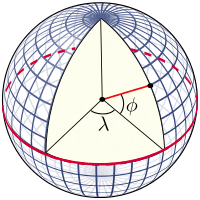A perspective view of the Earth showing how latitude (φ) and longitude (λ) are defined on a spherical model. The graticule spacing is 10 degrees.

### The graticule on the sphere

The graticule is formed by the lines of constant latitude and constant longitude, which are constructed with reference to the rotation axis of the Earth. The primary reference points are the poles where the axis of rotation of the Earth intersects the reference surface. Planes which contain the rotation axis intersect the surface at the meridians; and the angle between any one meridian plane and that through Greenwich (the Prime Meridian) defines the longitude: meridians are lines of constant longitude. The plane through the centre of the Earth and perpendicular to the rotation axis intersects the surface at a great circle called the Equator. Planes parallel to the equatorial plane intersect the surface in circles of constant latitude; these are the parallels. The Equator has a latitude of 0°, the North Pole has a latitude of 90° North (written 90° N or +90°), and the South Pole has a latitude of 90° South (written 90° S or −90°). The latitude of an arbitrary point is the angle between the equatorial plane and the radius to that point.

The latitude, as defined in this way for the sphere, is often termed the spherical latitude, to avoid ambiguity with auxiliary latitudes defined in subsequent sections of this article.

### Named latitudes on the Earth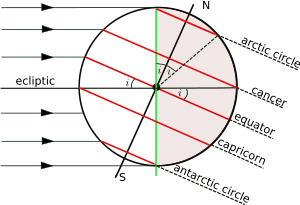The orientation of the Earth at the December solstice.

Besides the equator, four other parallels are of significance:

 Arctic Circle 66° 34′ (66.57°) N Tropic of Cancer 23° 26′ (23.43°) N Tropic of Capricorn 23° 26′ (23.43°) S Antarctic Circle 66° 34′ (66.57°) S

The plane of the Earth's orbit about the Sun is called the ecliptic, and the plane perpendicular to the rotation axis of the Earth is the equatorial plane. The angle between the ecliptic and the equatorial plane is called variously the axial tilt, the obliquity, or the inclination of the ecliptic, and it is conventionally denoted by i. The latitude of the tropical circles is equal to i and the latitude of the polar circles is its complement (90° - i). The axis of rotation varies slowly over time and the values given here are those for the current epoch. The time variation is discussed more fully in the article on axial tilt.[lower-alpha 2]

The figure shows the geometry of a cross-section of the plane perpendicular to the ecliptic and through the centres of the Earth and the Sun at the December solstice when the Sun is overhead at some point of the Tropic of Capricorn. The south polar latitudes below the Antarctic Circle are in daylight, whilst the north polar latitudes above the Arctic Circle are in night. The situation is reversed at the June solstice, when the Sun is overhead at the Tropic of Cancer. Only at latitudes in between the two tropics is it possible for the Sun to be directly overhead (at the zenith).

On map projections there is no universal rule as to how meridians and parallels should appear. The examples below show the named parallels (as red lines) on the commonly used Mercator projection and the Transverse Mercator projection. On the former the parallels are horizontal and the meridians are vertical, whereas on the latter there is no exact relationship of parallels and meridians with horizontal and vertical: both are complicated curves.

Normal Mercator Transverse Mercator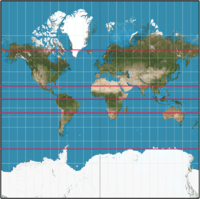\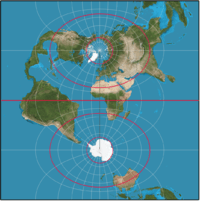### Meridian distance on the sphere

On the sphere the normal passes through the centre and the latitude (φ) is therefore equal to the angle subtended at the centre by the meridian arc from the equator to the point concerned. If the meridian distance is denoted by m(φ) thenwhere R denotes the mean radius of the Earth. R is equal to 6,371 km or 3,959 miles. No higher accuracy is appropriate for R since higher-precision results necessitate an ellipsoid model. With this value for R the meridian length of 1 degree of latitude on the sphere is 111.2 km or 69.1 miles. The length of 1 minute of latitude is 1.853 km or 1.151 miles, used as the basis of the nautical mile.

## Latitude on the ellipsoid

### Ellipsoids

In 1687 Isaac Newton published the Philosophiæ Naturalis Principia Mathematica, in which he proved that a rotating self-gravitating fluid body in equilibrium takes the form of an oblate ellipsoid. (This article uses the term ellipsoid in preference to the older term spheroid.) Newton's result was confirmed by geodetic measurements in the 18th century. (See Meridian arc.) An oblate ellipsoid is the three-dimensional surface generated by the rotation of an ellipse about its shorter axis (minor axis). "Oblate ellipsoid of revolution" is abbreviated to 'ellipsoid' in the remainder of this article. (Ellipsoids which do not have an axis of symmetry are termed triaxial.)

Many different reference ellipsoids have been used in the history of geodesy. In pre-satellite days they were devised to give a good fit to the geoid over the limited area of a survey but, with the advent of GPS, it has become natural to use reference ellipsoids (such as WGS84) with centre at the centre of mass of the Earth and minor axis aligned to the rotation axis of the Earth. These geocentric ellipsoids are usually within 100 m (330 ft) of the geoid. Since latitude is defined with respect to an ellipsoid, the position of a given point is different on each ellipsoid: one cannot exactly specify the latitude and longitude of a geographical feature without specifying the ellipsoid used. Many maps maintained by national agencies are based on older ellipsoids, so one must know how the latitude and longitude values are transformed from one ellipsoid to another. GPS handsets include software to carry out datum transformations which link WGS84 to the local reference ellipsoid with its associated grid.

### The geometry of the ellipsoid

The shape of an ellipsoid of revolution is determined by the shape of the ellipse which is rotated about its minor (shorter) axis. Two parameters are required. One is invariably the equatorial radius, which is the semi-major axis, a. The other parameter is usually (1) the polar radius or semi-minor axis, b; or (2) the (first) flattening, f; or (3) the eccentricity, e. These parameters are not independent: they are related byMany other parameters (see ellipse, ellipsoid) appear in the study of geodesy, geophysics and map projections but they can all be expressed in terms of one or two members of the set a, b, f and e. Both f and e are small and often appear in series expansions in calculations; they are of the order 1/300 and 0.08 respectively. Values for a number of ellipsoids are given in Figure of the Earth. Reference ellipsoids are usually defined by the semi-major axis and the inverse flattening, 1/f. For example, the defining values for the WGS84 ellipsoid, used by all GPS devices, are

• a (equatorial radius): 6378137.0 m exactly
• 1/f (inverse flattening): 298.257223563 exactly

from which are derived

• b (polar radius): 6356752.3142 m
• e2 (eccentricity squared): 0.00669437999014

The difference between the semi-major and semi-minor axes is about 21 km (13 miles) and as fraction of the semi-major axis it equals the flattening; on a computer the ellipsoid could be sized as 300 by 299 pixels. This would barely be distinguishable from a 300-by-300-pixel sphere, so illustrations usually exaggerate the flattening.

### Geodetic and geocentric latitudes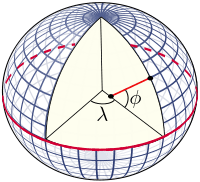The definition of geodetic latitude (φ) and longitude (λ) on an ellipsoid. The normal to the surface does not pass through the centre, except at the equator and at the poles.

The graticule on the ellipsoid is constructed in exactly the same way as on the sphere. The normal at a point on the surface of an ellipsoid does not pass through the centre, except for points on the equator or at the poles, but the definition of latitude remains unchanged as the angle between the normal and the equatorial plane. The terminology for latitude must be made more precise by distinguishing:

• Geodetic latitude: the angle between the normal and the equatorial plane. The standard notation in English publications is φ. This is the definition assumed when the word latitude is used without qualification. The definition must be accompanied with a specification of the ellipsoid.
• Geocentric latitude: the angle between the radius (from centre to the point on the surface) and the equatorial plane. (Figure below). There is no standard notation: examples from various texts include ψ, q, φ′, φc, φg. This article uses ψ.
• Spherical latitude: the angle between the normal to a spherical reference surface and the equatorial plane.
• Geographic latitude must be used with care. Some authors use it as a synonym for geodetic latitude whilst others use it as an alternative to the astronomical latitude.
• Latitude (unqualified) should normally refer to the geodetic latitude.

The importance of specifying the reference datum may be illustrated by a simple example. On the reference ellipsoid for WGS84, the centre of the Eiffel Tower has a geodetic latitude of 48° 51′ 29″ N, or 48.8583° N and longitude of 2° 17′ 40″ E or 2.2944°E. The same coordinates on the datum ED50 define a point on the ground which is 140 metres (460 feet) distant from the tower. A web search may produce several different values for the latitude of the tower; the reference ellipsoid is rarely specified.

### Length of a degree of latitude

Main article: Meridian arc

In Meridian arc and standard texts it is shown that the distance along a meridian from latitude φ to the equator is given by (φ in radians)where M(φ) is the meridional radius of curvature.

The distance from the equator to the pole isFor WGS84 this distance is 10001.965729 km.

The evaluation of the meridian distance integral is central to many studies in geodesy and map projection. It can be evaluated by expanding the integral by the binomial series and integrating term by term: see Meridian arc for details. The length of the meridian arc between two given latitudes is given by replacing the limits of the integral by the latitudes concerned. The length of a small meridian arc is given byφΔ1
lat
Δ1
long
110.574 km 111.320 km
15° 110.649 km 107.550 km
30° 110.852 km 96.486 km
45° 111.132 km 78.847 km
60° 111.412 km 55.800 km
75° 111.618 km 28.902 km
90° 111.694 km 0.000 km

When the latitude difference is 1 degree, corresponding to π/180 radians, the arc distance is aboutThe distance in metres (correct to 0.01 metre) between latitudes φ  0.5 degrees and φ + 0.5 degrees on the WGS84 spheroid isThe variation of this distance with latitude (on WGS84) is shown in the table along with the length of a degree of longitude (east-west distance):A calculator for any latitude is provided by the U.S. Government's National Geospatial-Intelligence Agency (NGA).

Historically a nautical mile was defined as the length of one minute of arc along a meridian of a spherical earth. An ellipsoid model leads to a variation of the nautical mile with latitude. This was resolved by defining the nautical mile to be exactly 1,852 metres.

## Auxiliary latitudes

There are six auxiliary latitudes that have applications to special problems in geodesy, geophysics and the theory of map projections:

• Geocentric latitude
• Reduced (or parametric) latitude
• Rectifying latitude
• Authalic latitude
• Conformal latitude
• Isometric latitude

The definitions given in this section all relate to locations on the reference ellipsoid but the first two auxiliary latitudes, like the geodetic latitude, can be extended to define a three-dimensional geographic coordinate system as discussed below. The remaining latitudes are not used in this way; they are used only as intermediate constructs in map projections of the reference ellipsoid to the plane or in calculations of geodesics on the ellipsoid. Their numerical values are not of interest. For example, no one would need to calculate the authalic latitude of the Eiffel Tower.

The expressions below give the auxiliary latitudes in terms of the geodetic latitude, the semi-major axis, a, and the eccentricity, e. (For inverses see below.) The forms given are, apart from notational variants, those in the standard reference for map projections, namely "Map projections: a working manual" by J. P. Snyder. Derivations of these expressions may be found in Adams and online publications by Osborne and Rapp.

### Geocentric latitude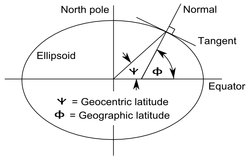The definition of geodetic (or geographic) and geocentric latitudes.

The geocentric latitude is the angle between the equatorial plane and the radius from the centre to a point on the surface. The relation between the geocentric latitude (ψ) and the geodetic latitude (φ) is derived in the above references asThe geodetic and geocentric latitudes are equal at the equator and at the poles. The value of the squared eccentricity is approximately 0.0067 (depending on the choice of ellipsoid) and the maximum difference, φψ, is about 11.5 minutes of arc at a geodetic latitude of 45° 5′.

### Reduced (or parametric) latitude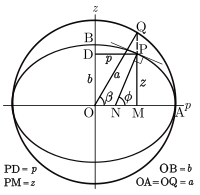Definition of the reduced latitude (β) on the ellipsoid.

The reduced or parametric latitude, β, is defined by the radius drawn from the centre of the ellipsoid to that point Q on the surrounding sphere (of radius a) which is the projection parallel to the Earth's axis of a point P on the ellipsoid at latitude φ. It was introduced by Legendre and Bessel who solved problems for geodesics on the ellipsoid by transforming them to an equivalent problem for spherical geodesics by using this smaller latitude. Bessel's notation, u(φ), is also used in the current literature. The reduced latitude is related to the geodetic latitude by:The alternative name arises from the parameterization of the equation of the ellipse describing a meridian section. In terms of Cartesian coordinates p, the distance from the minor axis, and z, the distance above the equatorial plane, the equation of the ellipse is:The Cartesian coordinates of the point are parameterized byCayley suggested the term parametric latitude because of the form of these equations.

The reduced latitude is not used in the theory of map projections. Its most important application is in the theory of ellipsoid geodesics. (Vincenty, Karney).

### Rectifying latitude

The rectifying latitude, μ, is the meridian distance scaled so that its value at the poles is equal to 90 degrees or π/2 radians:where the meridian distance from the equator to a latitude φ is (see Meridian arc)and the length of the meridian quadrant from the equator to the pole (the polar distance) isUsing the rectifying latitude to define a latitude on a sphere of radiusdefines a projection from the ellipsoid to the sphere such that all meridians have true length and uniform scale. The sphere may then be projected to the plane with an equirectangular projection to give a double projection from the ellipsoid to the plane such that all meridians have true length and uniform meridian scale. An example of the use of the rectifying latitude is the Equidistant conic projection. (Snyder, Section 16). The rectifying latitude is also of great importance in the construction of the Transverse Mercator projection.

### Authalic latitude

The authalic (Greek for same area) latitude, ξ, gives an area-preserving transformation to a sphere.whereandand the radius of the sphere is taken asAn example of the use of the authalic latitude is the Albers equal-area conic projection.:§14

### Conformal latitude

The conformal latitude, χ, gives an angle-preserving (conformal) transformation to the sphere.where gd(x) is the Gudermannian function. (See also Mercator projection.) The conformal latitude defines a transformation from the ellipsoid to a sphere of arbitrary radius such that the angle of intersection between any two lines on the ellipsoid is the same as the corresponding angle on the sphere (so that the shape of small elements is well preserved). A further conformal transformation from the sphere to the plane gives a conformal double projection from the ellipsoid to the plane. This is not the only way of generating such a conformal projection. For example, the 'exact' version of the Transverse Mercator projection on the ellipsoid is not a double projection. (It does, however, involve a generalisation of the conformal latitude to the complex plane).

### Isometric latitude

The isometric latitude is conventionally denoted by ψ (not to be confused with the geocentric latitude): it is used in the development of the ellipsoidal versions of the normal Mercator projection and the Transverse Mercator projection. The name "isometric" arises from the fact that at any point on the ellipsoid equal increments of ψ and longitude λ give rise to equal distance displacements along the meridians and parallels respectively. The graticule defined by the lines of constant ψ and constant λ, divides the surface of the ellipsoid into a mesh of squares (of varying size). The isometric latitude is zero at the equator but rapidly diverges from the geodetic latitude, tending to infinity at the poles. The conventional notation is given in Snyder (page 15):For the normal Mercator projection (on the ellipsoid) this function defines the spacing of the parallels: if the length of the equator on the projection is E (units of length or pixels) then the distance, y, of a parallel of latitude φ from the equator isThe isometric latitude ψ is closely related to the conformal latitude χ:### Inverse formulae and series

The formulae in the previous sections give the auxiliary latitude in terms of the geodetic latitude. The expressions for the geocentric and reduced latitudes may be inverted directly but this is impossible in the four remaining cases: the rectifying, authalic, conformal, and isometric latitudes. There are two methods of proceeding. The first is a numerical inversion of the defining equation for each and every particular value of the auxiliary latitude. The methods available are fixed-point iteration and Newton–Raphson root finding. The other, more useful, approach is to express the auxiliary latitude as a series in terms of the geodetic latitude and then invert the series by the method of Lagrange reversion. Such series are presented by Adams who uses Taylor series expansions and gives coefficients in terms of the eccentricity. Osborne derives series to arbitrary order by using the computer algebra package Maxima and expresses the coefficients in terms of both eccentricity and flattening. The series method is not applicable to the isometric latitude and one must use the conformal latitude in an intermediate step.

### Numerical comparison of auxiliary latitudes

The following plot shows the magnitude of the difference between the geodetic latitude, (denoted as the "common" latitude on the plot), and the auxiliary latitudes other than the isometric latitude (which diverges to infinity at the poles). In every case the geodetic latitude is the greater. The differences shown on the plot are in arc minutes. The horizontal resolution of the plot fails to make clear that the maxima of the curves are not at 45° but calculation shows that they are within a few arc minutes of 45°. Some representative data points are given in the table following the plot. Note the closeness of the conformal and geocentric latitudes. This was exploited in the days of hand calculators to expedite the construction of map projections.:108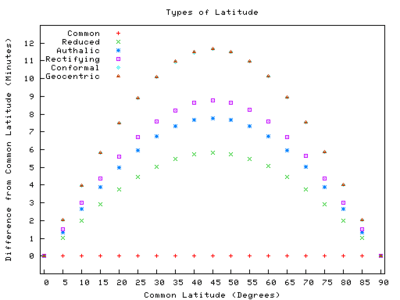Approximate difference from geodetic latitude (φ)
φ Reduced
φβ
Authalic
φξ
Rectifying
φμ
Conformal
φχ
Geocentric
φψ
0.00′ 0.00′ 0.00′ 0.00′ 0.00′
15° 2.91′ 3.89′ 4.37′ 5.82′ 5.82′
30° 5.05′ 6.73′ 7.57′10.09′10.09′
45° 5.84′ 7.78′ 8.76′11.67′11.67′
60° 5.06′ 6.75′ 7.59′10.12′10.13′
75° 2.92′ 3.90′ 4.39′ 5.85′ 5.85′
90° 0.00′ 0.00′ 0.00′ 0.00′ 0.00′

## Latitude and coordinate systems

The geodetic latitude, or any of the auxiliary latitudes defined on the reference ellipsoid, constitutes with longitude a two-dimensional coordinate system on that ellipsoid. To define the position of an arbitrary point it is necessary to extend such a coordinate system into three dimensions. Three latitudes are used in this way: the geodetic, geocentric and reduced latitudes are used in geodetic coordinates, spherical polar coordinates and ellipsoidal coordinates respectively.

### Geodetic coordinates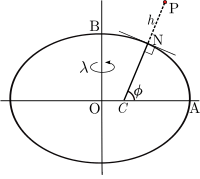Geodetic coordinates P(ɸ,λ,h)

At an arbitrary point P consider the line PN which is normal to the reference ellipsoid. The geodetic coordinates P(ɸ,λ,h) are the latitude and longitude of the point N on the ellipsoid and the distance PN. This height differs from the height above the geoid or a reference height such as that above mean sea level at a specified location. The direction of PN will also differ from the direction of a vertical plumb line. The relation of these different heights requires knowledge of the shape of the geoid and also the gravity field of the Earth.

### Spherical polar coordinates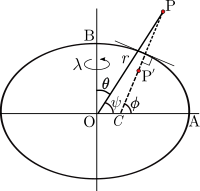Geocentric coordinate related to spherical polar coordinates P(r,θ,λ)

The geocentric latitude ψ is the complement of the polar angle θ in conventional spherical polar coordinates in which the coordinates of a point are P(r,θ,λ) where r is the distance of P from the centre O, θ is the angle between the radius vector and the polar axis and λ is longitude. Since the normal at a general point on the ellipsoid does not pass through the centre it is clear that points on the normal, which all have the same geodetic latitude, will have differing geocentric latitudes. Spherical polar coordinate systems are used in the analysis of the gravity field.

### Ellipsoidal coordinates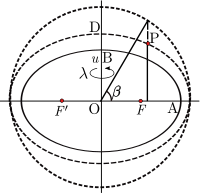Ellipsoidal coordinates P(u,β,λ)

The reduced latitude can also be extended to a three-dimensional coordinate system. For a point P not on the reference ellipsoid (semi-axes OA and OB) construct an auxiliary ellipsoid which is confocal (same foci F, F′) with the reference ellipsoid: the necessary condition is that the product ae of semi-major axis and eccentricity is the same for both ellipsoids. Let u be the semi-minor axis (OD) of the auxiliary ellipsoid. Further let β be the reduced latitude of P on the auxiliary ellipsoid. The set (u,β,λ) define the ellipsoid coordinates.:§4.2.2 These coordinates are the natural choice in models of the gravity field for a uniform distribution of mass bounded by the reference ellipsoid.

### Coordinate conversions

The relations between the above coordinate systems, and also Cartesian coordinates are not presented here. The transformation between geodetic and Cartesian coordinates may be found in Geographic coordinate conversion. The relation of Cartesian and spherical polars is given in Spherical coordinate system. The relation of Cartesian and ellipsoidal coordinates is discussed in Torge.

## Astronomical latitude

Astronomical latitude (Φ) is the angle between the equatorial plane and the true vertical at a point on the surface. The true vertical, the direction of a plumb line, is also the direction of the gravity acceleration, the resultant of the gravitational acceleration (mass-based) and the centrifugal acceleration at that latitude. Astronomic latitude is calculated from angles measured between the zenith and stars whose declination is accurately known.

In general the true vertical at a point on the surface does not exactly coincide with either the normal to the reference ellipsoid or the normal to the geoid. The angle between the astronomic and geodetic normals is usually a few seconds of arc but it is important in geodesy. The reason why it differs from the normal to the geoid is, because the geoid is an idealized, theoretical shape "at mean sea level". Points on the real surface of the earth are usually above or below this idealized geoid surface and here the true vertical can vary slightly. Also, the true vertical at a point at a specific time is influenced by tidal forces, which the theoretical geoid averages out.

Astronomical latitude is not to be confused with declination, the coordinate astronomers use in a similar way to specify the angular position of stars north/south of the celestial equator (see equatorial coordinates), nor with ecliptic latitude, the coordinate that astronomers use to specify the angular position of stars north/south of the ecliptic (see ecliptic coordinates).

## Notes

1. The current full documentation of ISO 19111 may be purchased from http://www.iso.org but drafts of the final standard are freely available at many web sites, one such is available at the following CSIRO
2. The value of this angle today is 23°26′13.5″ (or 23.43709°). This figure is provided by Template:Circle of latitude.

## References

1. Torge, W. (2001). Geodesy (3rd ed.). De Gruyter. ISBN 3-11-017072-8.
2. Hofmann-Wellenhof, B.; Moritz, H. (2006). Physical Geodesy (2nd ed.). ISBN 3-211-33544-7.
3. Semedo de Matos, Jorge (2015). "Tábuas Solares na náutica portuguesa dos séculos XV e XVI". In Contente Domingues, Francisco. D’Aquém, d’Além e d’Ultramar. Homenagem a António Dias Farinha. Lisboa: CHUL. pp. 1235–1250.
4. Newton, Isaac. "Book III Proposition XIX Problem III". Philosophiæ Naturalis Principia Mathematica. Translated by Motte, Andrew. p. 407.
5. "TR8350.2". National Geospatial-Intelligence Agency publication. p. 3-1.
6. Osborne, Peter (2013). "Chapters 5,6". The Mercator Projections. doi:10.5281/zenodo.35392. doi:10.5281/zenodo.35562 for LaTeX code and figures.
7. Rapp, Richard H. (1991). "Chapter 3". Geometric Geodesy, Part I. Columbus, OH: Dept. of Geodetic Science and Surveying, Ohio State Univ.
8. "Length of degree calculator". National Geospatial-Intelligence Agency.
9. Snyder, John P. (1987). Map Projections: A Working Manual. U.S. Geological Survey Professional Paper 1395. Washington, DC: United States Government Printing Office.
10. Adams, Oscar S. (1921). Latitude Developments Connected With Geodesy and Cartography (with tables, including a table for Lambert equal area meridional projection (PDF). Special Publication No. 67. US Coast and Geodetic Survey. Warning: Adams uses the nomenclature isometric latitude for the conformal latitude of this article (and throughout the modern literature).
11. Legendre, A. M. (1806). "Analyse des triangles tracés sur la surface d'un sphéroïde". Mém. Inst. Nat. Fr. 1st semester: 130–161.
12. Bessel, F. W. (1825). "Über die Berechnung der geographischen Langen und Breiten aus geodatischen Vermessungen". Astron. Nachr. 4 (86): 241–254. doi:10.1002/asna.201011352.
Translation: Karney, C. F. F.; Deakin, R. E. (2010). "The calculation of longitude and latitude from geodesic measurements". Astron. Nachr. 331 (8): 852–861. arXiv:0908.1824. Bibcode:1825AN......4..241B.
13. Cayley, A. (1870). "On the geodesic lines on an oblate spheroid". Phil. Mag. 40 (4th ser.): 329–340.
14. Karney, C. F. F. (2013). "Algorithms for geodesics". J. Geodesy. 87 (1): 43–55. doi:10.1007/s00190-012-0578-z.